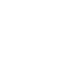# ACT & SAT Question of the Day: Feb. 15, 2014

If you are reading this in an email you received from me, do not click the link to sat.collegeboard.org below. Use the link to my website that is farther down on the email.

http://sat.collegeboard.org/practice/sat-question-of-the-day?questionId=20140215&oq=1 (This link takes you to today’s question. If you use my archive, you will see the question related to my SAT explanation for that date.)

This is a tough question that only 37% of students are getting correct.  It would definitely show up late in a math section because the questions are arranged from easy to hard.  Have you set a target score?  Do you even need to bother doing this question?  If so, how important is it for you to get it correct?  Watch what I have to say about target scores on Video #105.

The answer is D.  You can do this question a couple of ways.  First, you could do it if you recognize and understand equations for parabolas.  This is a downward-facing parabola (because the coefficient of p-squared is negative).  They tell you the range is from 0 to 200.  Therefore, the peak of the parabola is half way between the two extremes, 100 or Answer D.  No fuss, no muss, no calculations!  You just need to think!

Second, you could just plug in the answers for p and turn the math crank.  That would take a while using your calculator.  But there’s a way to eliminate some of the answers without doing any work.  Always start in the middle with answer C.  Then try D.  You would see the graph is going up at that point which would eliminate answers A and B without doing any work!  Then when you try Answer E you realize it started going down after 100; so pick D.

Use this question to help realize how much the SAT is truly a “Reasoning Test.”  You could have done this question without any calculations by applying your knowledge about parabolas.  If you had to do calculations, using the structure of the test (Pillar I) would have saved you some time.  In either case, you can see that this test is more than about the strength of your math skills.

Let’s see what the ACT folks have in store for us today.

Shame on me for not having a system for finding my previous blogs based on the ACT questions.  I just wrote about this one a couple of weeks ago and here it is again.  It was even so recent that I remember the answer without doing any calculations.  That’s no fun.  I wish the ACT folks would include some new questions.

The answer is F.  Let’s analyze the formula.  The area of a trapezoid is the height times the average of the parallel bases (1/2 times b1 + b2) .  Doing a little quick arithmetic, you can plug in the measurements they give you and turn the math crank.  The area, 55, divided by by the height, 5 equals 11 which is the average of the parallel bases.  One base is 13; so the other base has to be 9 because the average of 9 and 13 is 11.  Use my “balancing point” strategy to figure out the short side is 9 without doing any calculations other than subtracting!  (Watch the math tip video on my website home page.)

I like it!!  You are spending some of your Saturday doing some SAT/ACT prep.  Congrats.  You deserve a pat on the back.

The SAT & ACT Wizard